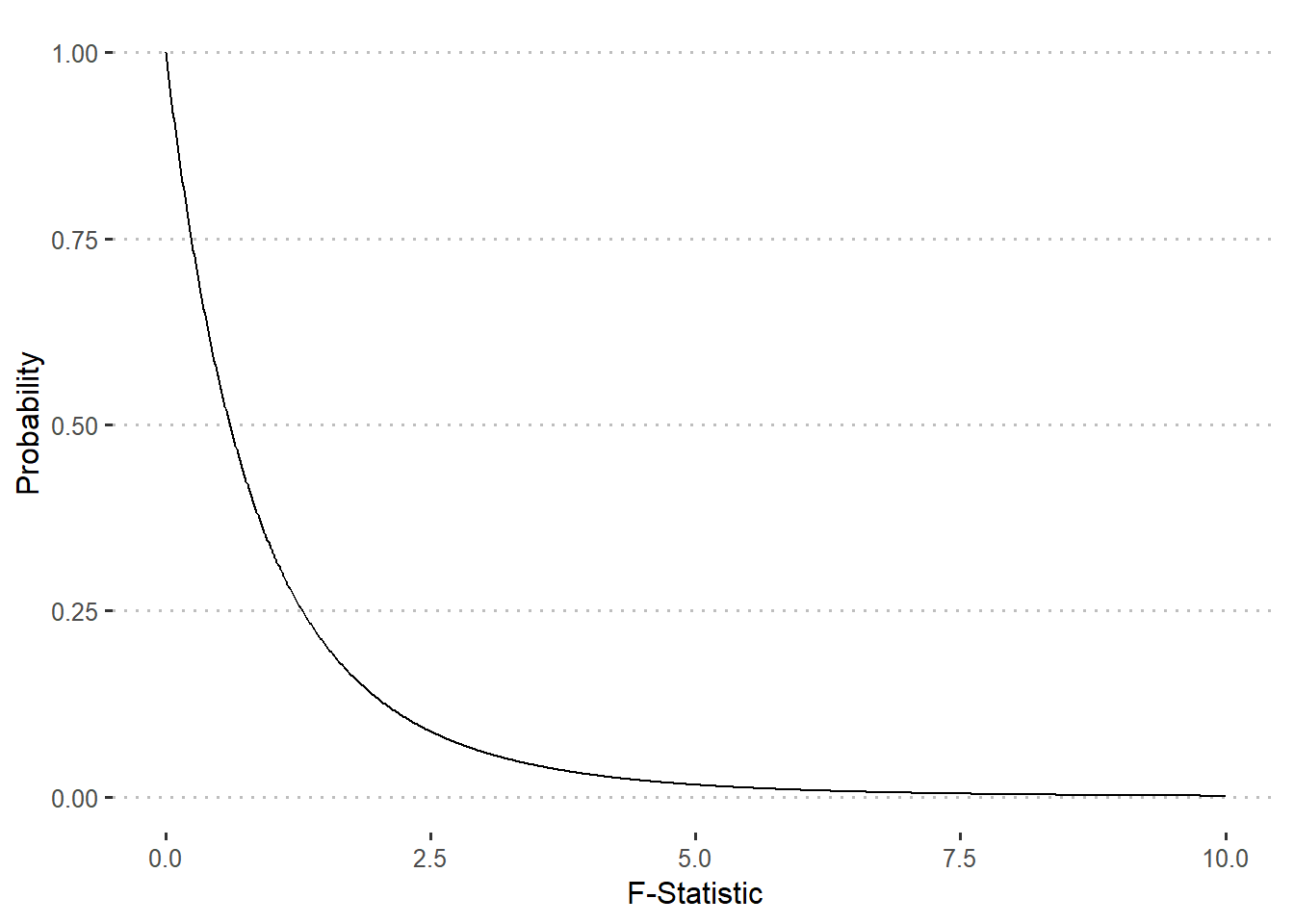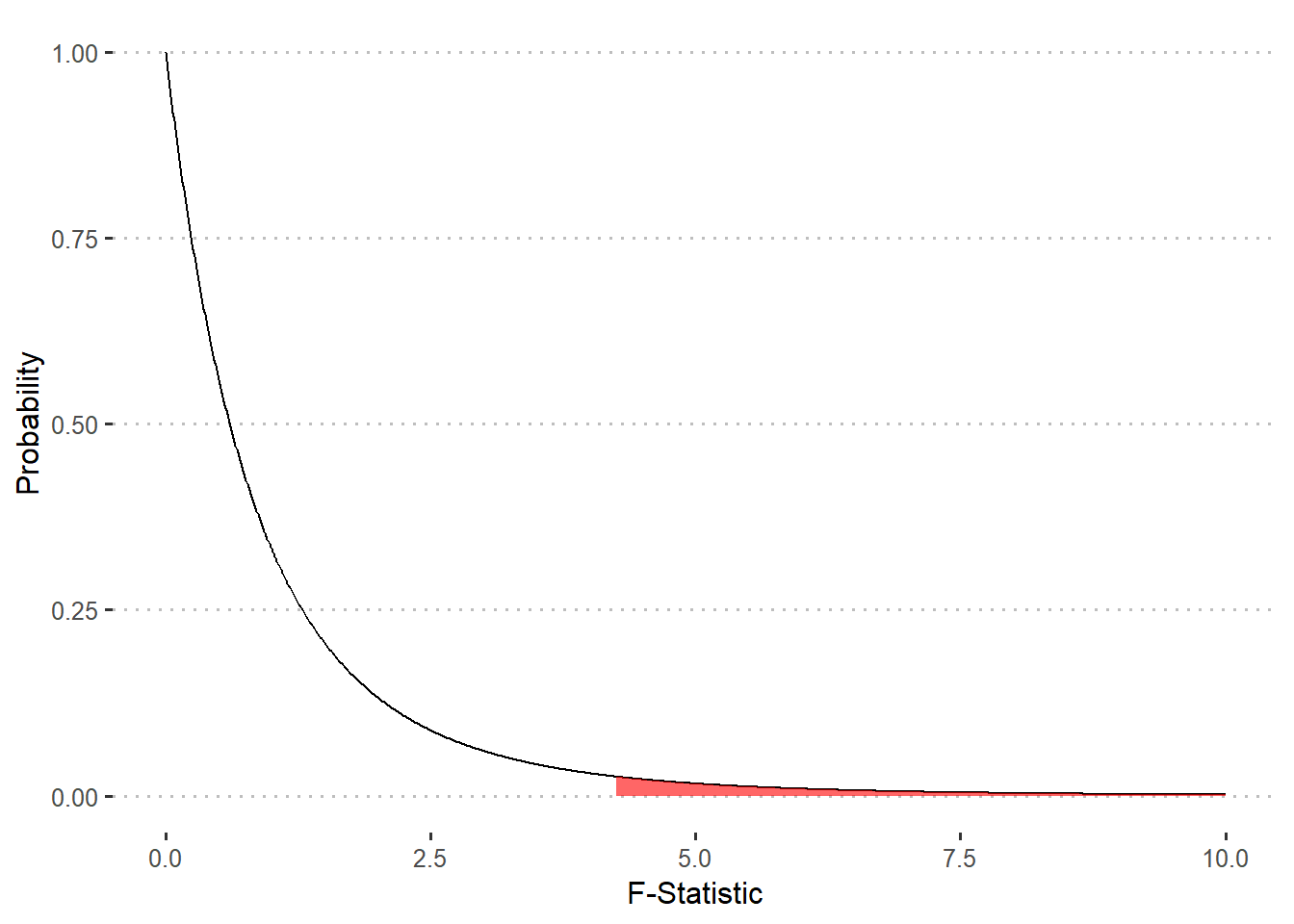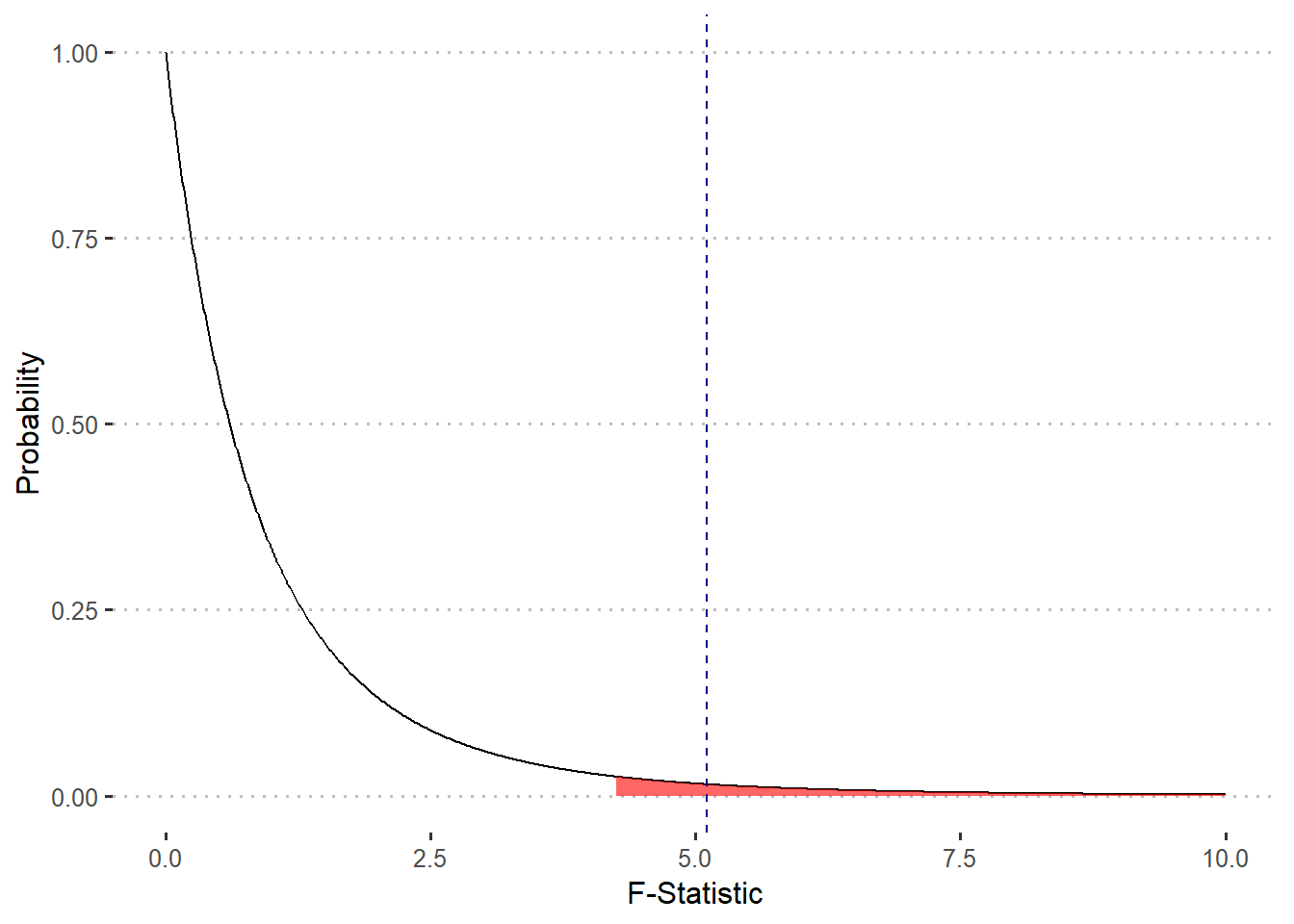## Upcoming Assignments/Quizzes

Assignments Open Time Due Time
ANOVA Article Analysis Activity October 22nd (1:00 am EST) October 28th (11:55 pm EST)
Module 8 Data Quiz October 26th (1:00 am EST) October 28th (11:55 pm EST)
Module 9 Conceptual Quiz October 26th (1:00 am EST) October 28th (11:55 pm EST)

## Notes from Discussion Board/Office Hours

### Relationship between the $$F$$-statistic, p-value, and null hypothesis

In sub-module 9.3, Dr. Baiser covers how to test hypotheses using ANOVA. To do this, we calculate our observed $$F$$-statistic using the mean square among groups and mean square within group from our observed data, and compare that to the distribution of possible $$F$$-statistics (i.e. the $$F$$ distribution) based on the degrees of freedom (df) in the numeration and denominator of our $$F$$-statistic to determine how significance of our observed value.

Let’s make some plots to visualize this comparison step-by-step. I’ll use the same example from the sub-module 9.3 lecture. Let’s start from when we calculate our observed $$F$$-statistics (pg. 15 from 9.3 notes), which I’ll call f_obs. Based on our calculations of the mean squares we determined that $$F_{obs} = 5.11$$.

Now let’s draw our $$F$$-distribution. Recall that this is determined by the dfs in the numerator ($$df_{num}$$) and the denominator ($$df_{den}$$) of our $$F$$-statistic. If we have $$a$$ number of treatments and $$n$$ number of replicates, than $$df_{num} = a - 1$$ and $$df_{den} = n(a-1)$$. In our example, $$a=3$$ and $$n=4$$ (pg. 8), therefore $$df_{num} = 2$$ and $$df_{num} = 9$$. With this information we can draw our $$F$$-distribution by creating a vector of possible values of $$F$$ and passing those into the df() function in .

library(tidyverse)
library(ggpubr)

# Possible values of F-stat:
x = seq(from = 0, to = 10, by = 0.01)

# Probability of possible values of F-stat
y = df(x = x, df1 = 2, df2 = 9)

ggplot() +
geom_line(aes(x, y)) +
labs(x = "F-Statistic", y = "Probability") +
theme_pubclean()This curve shows the possible values for the $$F$$-statistic (shown on the x-axis) and the probability of observing those values (y-axis) if the null hypothesis were true (based on the dfs we specified). We can use this to determine if we should reject or fail to reject the null hypothesis by comparing f_obs to a theoretical $$F$$-statistic based on a critical value $$\alpha$$, which you’ll recall is often set to $$\alpha = 0.05$$. This $$F$$-statistic, which we will call f_crit, will correspond to having a p-value of exactly 0.05.

It is important to note that we working with a density function, which means that we are interested in the area under the curve. We can not simply draw a line with a y-intercept of 0.05 to find f_crit. Instead we need to find the “quantile” of our area of interest (5% or 0.05). Luckily the qf() can calculate quantile for the $$F$$-distribution:

f_crit <- qf(p = 0.05, df1 = 2, df2 = 9, lower.tail = F) 

Which determines that f_crit is equal to 4.26. Note that we set lower.tail = F because were are using a one-way test on the high end. Now we can draw the area under the curve that represents the “rejection region”:

ggplot(data.frame(x,y)) +
geom_line(aes(x, y)) +
stat_function(fun = df,
args = list(df1 = 2, df2 = 9),
xlim = c(f_crit, 10),
geom = "area",
fill = "red",
alpha = 0.6) +
labs(x = "F-Statistic", y = "Probability") +
theme_pubclean()Finally, let’s add f_obs to our plot:

f_obs = 5.11

ggplot(data.frame(x,y)) +
geom_line(aes(x, y)) +
stat_function(fun = df,
args = list(df1 = 2, df2 = 9),
xlim = c(f_crit, 10),
geom = "area",
fill = "red",
alpha = 0.6) +
geom_vline(aes(xintercept = f_obs), color = "darkblue", linetype = 2) +
labs(x = "F-Statistic", y = "Probability") +
theme_pubclean()As you can see, f_obs falls in the rejection region, and therefore we will reject the null hypothesis that there is no difference between our treatments. As a final note, we can also calculate the p-value associated with f_obs using the pf() function:

p_value <- pf(f_obs, df1 = 2, df2 = 9, lower.tail = F)
round(p_value, 3)
##  0.033